# Introduction

It's a little old article, but I read "How to get master data of stations and routes --Qiita" and learned that there is CSV data of routes. It was. Let's create a Tokyo subway map using the CSV file in "Station data.jp" introduced in this article. The Java version used is 14. Use yEd --Graph Editor to display the graph. All source code can be found here [https://gist.github.com/saka1029/7d08fef8744a508b29e1c8206510f21e).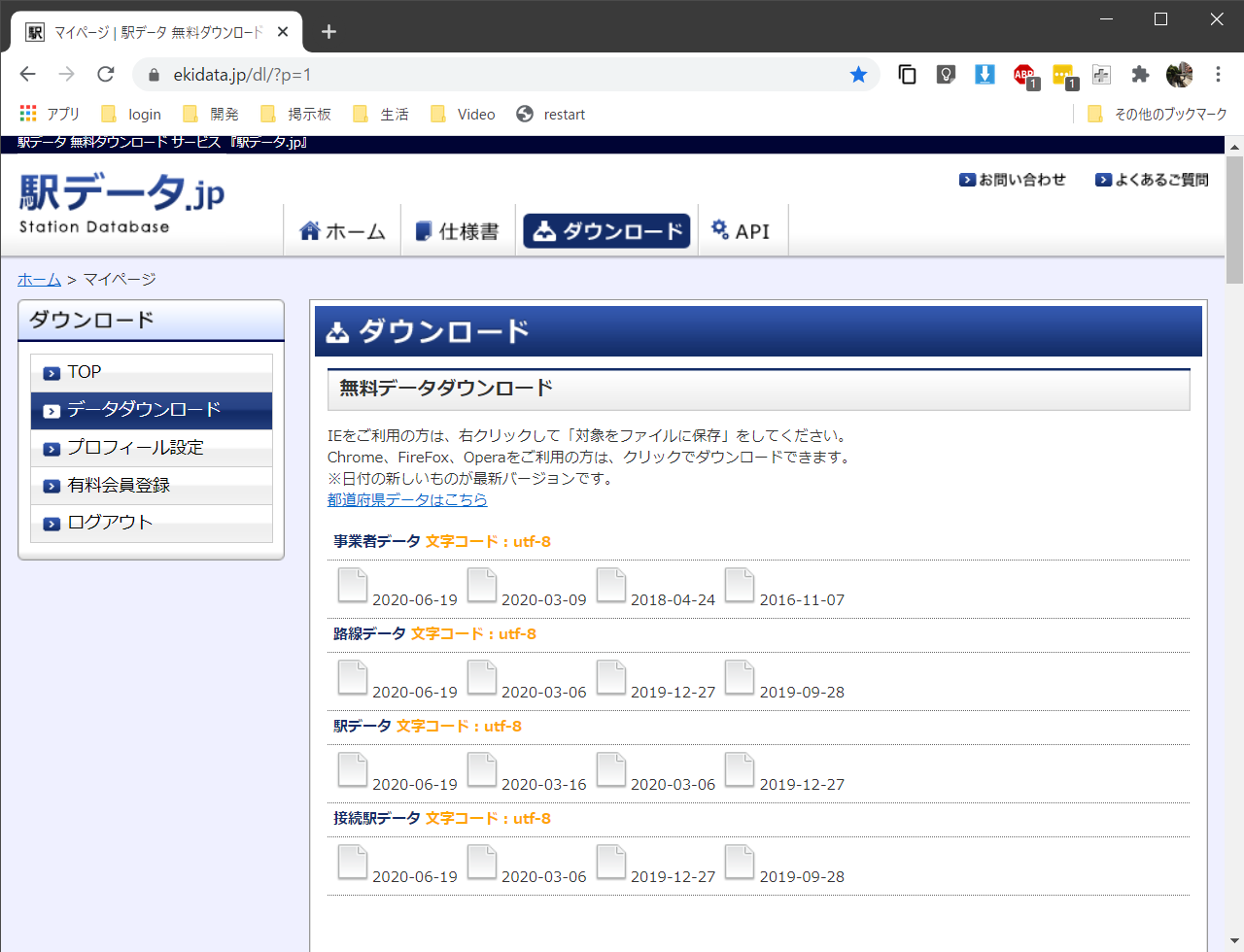Create a common program that reads a CSV file and converts it to `List <List <String >>`.

``````static final Charset CHARSET = StandardCharsets.UTF_8;
static final Path DIRECTORY = Paths.get("data", "eki");
static final Path LINE_CSV = DIRECTORY.resolve("line20200619free.csv");
static final Path STATION_CSV = DIRECTORY.resolve("station20200619free.csv");
static final Path JOIN_CSV = DIRECTORY.resolve("join20200619.csv");
static final Path GML = DIRECTORY.resolve("metro.gml");

static List<List<String>> readCSV(Path file) throws IOException {
.map(line -> List.of(line.split(",")))
.collect(toList());
}
``````

Each item is not enclosed in quotation marks, so it can be easily read.

First, read the route data. Since we will create a subway map of Tokyo, only those whose line names start with "Tokyo Metro" or "Toei" will be extracted.

``````//Tokyo subway line
.filter(line -> line.get(2).startsWith("Tokyo metro") || line.get(2).startsWith("Toei"))
.collect(toList());
``````

Next, the station data is read, but a list of line codes is created in order to extract only those that correspond to the line codes of the line data read earlier. (Should have been Set instead of List)

``````//List of Tokyo subway line codes
List<String> lineCodes = lines.stream()
.map(line -> line.get(0))
.collect(toList());
``````

Only the stations with the route codes in this list will be extracted.

``````//Tokyo subway station
.filter(station -> lineCodes.contains(station.get(5)))
.collect(toList());
``````

Finally, read the connection station data. Again, only those related to the Tokyo subway line code are extracted.

``````//Tokyo subway station connection
.filter(line -> lineCodes.contains(line.get(0)))
.collect(toList());
``````

# Creating a graph

Create a graph from the read data. Graphs are read using yEd --Graph Editor, so create text data in the format of GML (Graphic Modeling Language).

``````//Creating a graph
try (PrintWriter w = new PrintWriter(Files.newBufferedWriter(GML))) {
w.println("graph [");
for (List<String> s : stations) {
w.println("  node [");
w.println("    id " + s.get(0));     //Station code
w.println("    label \"" + s.get(2) + "\"");  //Station name
w.println("  ]");
}
for (List<String> j : joins) {
w.println("  edge [");
w.println("    source " + j.get(1));    //Connection station code 1
w.println("    target " + j.get(2));    //Connection station code 2
w.println("  ]");
}
w.println("]");
}
``````

Create a station as a node and a connecting station as an edge.

# First graph

Read with yEd and format as follows.

• Tools -> Fit Node to Label
Each node is displayed as a fixed size square, but the width of the node is variable to fit the size of the label text displayed inside the node.
• Layout -> Organic
Since each node has no location information, all nodes are displayed in the center of the screen. The Layout menu lays this out for easy viewing. We will use the Organic layout here.

Then, the graph looks like this. 13 routes have been created separately. You can see that the upper left is the Oedo line because it includes the loop line, and the Marunouchi line that includes the Honancho branch line because it is Y-shaped next to it.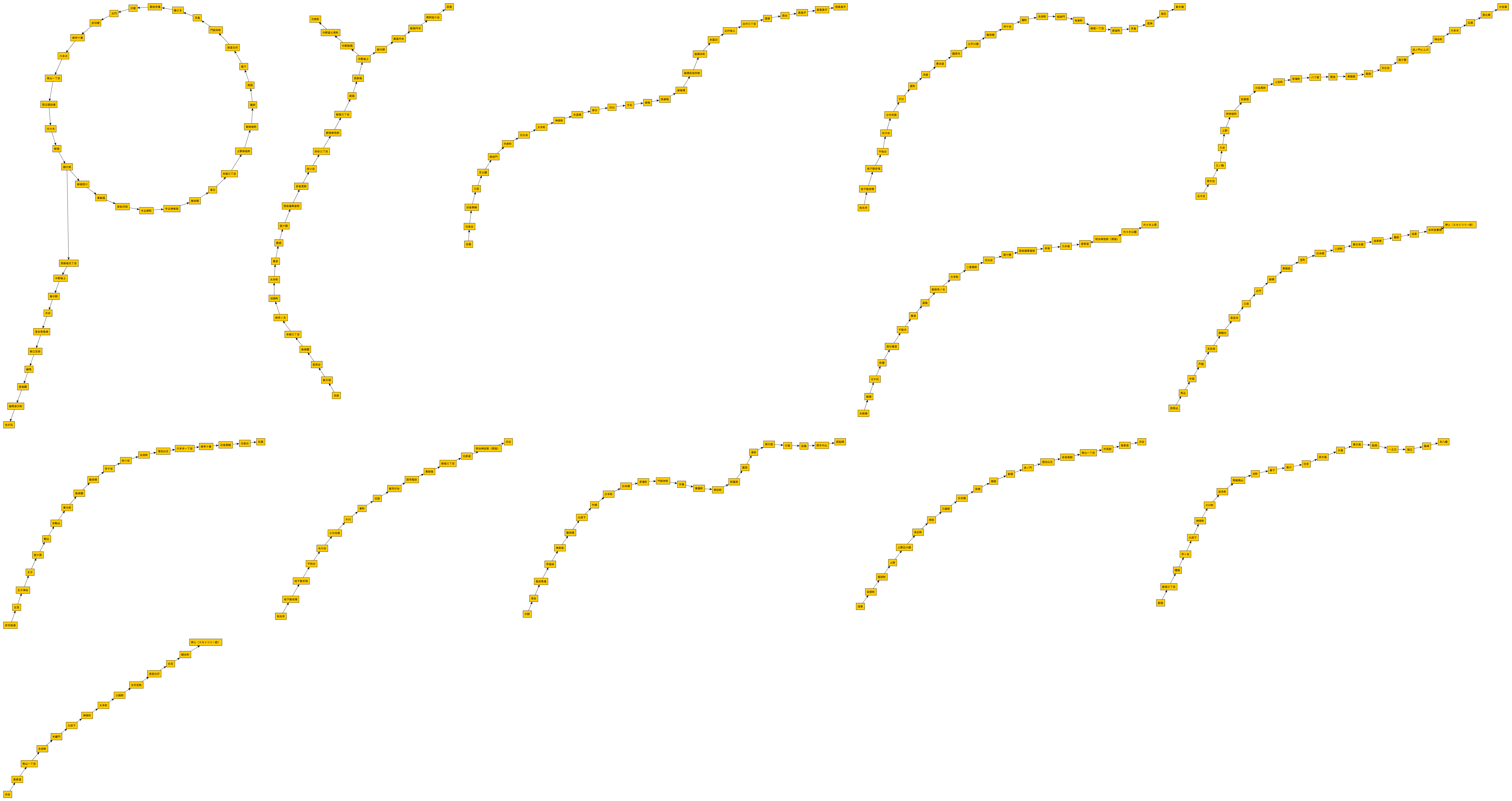However, this is not a route map. The reason for this is that the same station is registered separately for each line.

# Aggregated by the same station name

The station codes are aggregated by those with the same station name. The result is a map of the list, with the key being the station name and the value being the list of station codes with that station name. For example, `Shinjuku = [2800218, 9930128, 9930401]`.

``````//Station code grouped by station name(ex.Shinjuku=[2800218, 9930128, 9930401])
Map<String, List<String>> stationNameMap = stations.stream()
.collect(groupingBy(e -> e.get(2),
mapping(e -> e.get(0), toList())));
``````

In order to combine the codes representing Shinjuku into one, we will create a map to convert the station code to the representative station code. The station code that appears at the top of the station code list is the representative station code. In the case of Shinjuku, it will be `2800218 = 2800218, 9930128 = 2800218, 9930401 = 2800218`.

``````//Map from station code to representative station code(ex. 2800218=2800218, 9930128=2800218, 9930401=2800218)
Map<String, String> stationCodeMap = stationNameMap.values().stream()
.flatMap(codes -> codes.stream().map(code -> Map.entry(code, codes.get(0))))
.collect(toMap(Entry::getKey, Entry::getValue));
``````

# Creating a graph that aggregates the same station names

Create a graph that summarizes the same station names.

``````
//Creating a graph
try (PrintWriter w = new PrintWriter(Files.newBufferedWriter(GML))) {
w.println("graph [");
for (Entry<String, List<String>> e : stationNameMap.entrySet()) {   //Uses data aggregated by station name
w.println("  node [");
w.println("    id " + e.getValue().get(0));     //Representative station code
w.println("    label \"" + e.getKey() + "\"");  //Station name
w.println("  ]");
}
for (List<String> j : joins) {
w.println("  edge [");
w.println("    source " + stationCodeMap.get(j.get(1)));  //Convert to representative station code
w.println("    target " + stationCodeMap.get(j.get(2)));  //Convert to representative station code
w.println("  ]");
}
w.println("]");
}
``````

When I read it with yEd and format it, it looks like this.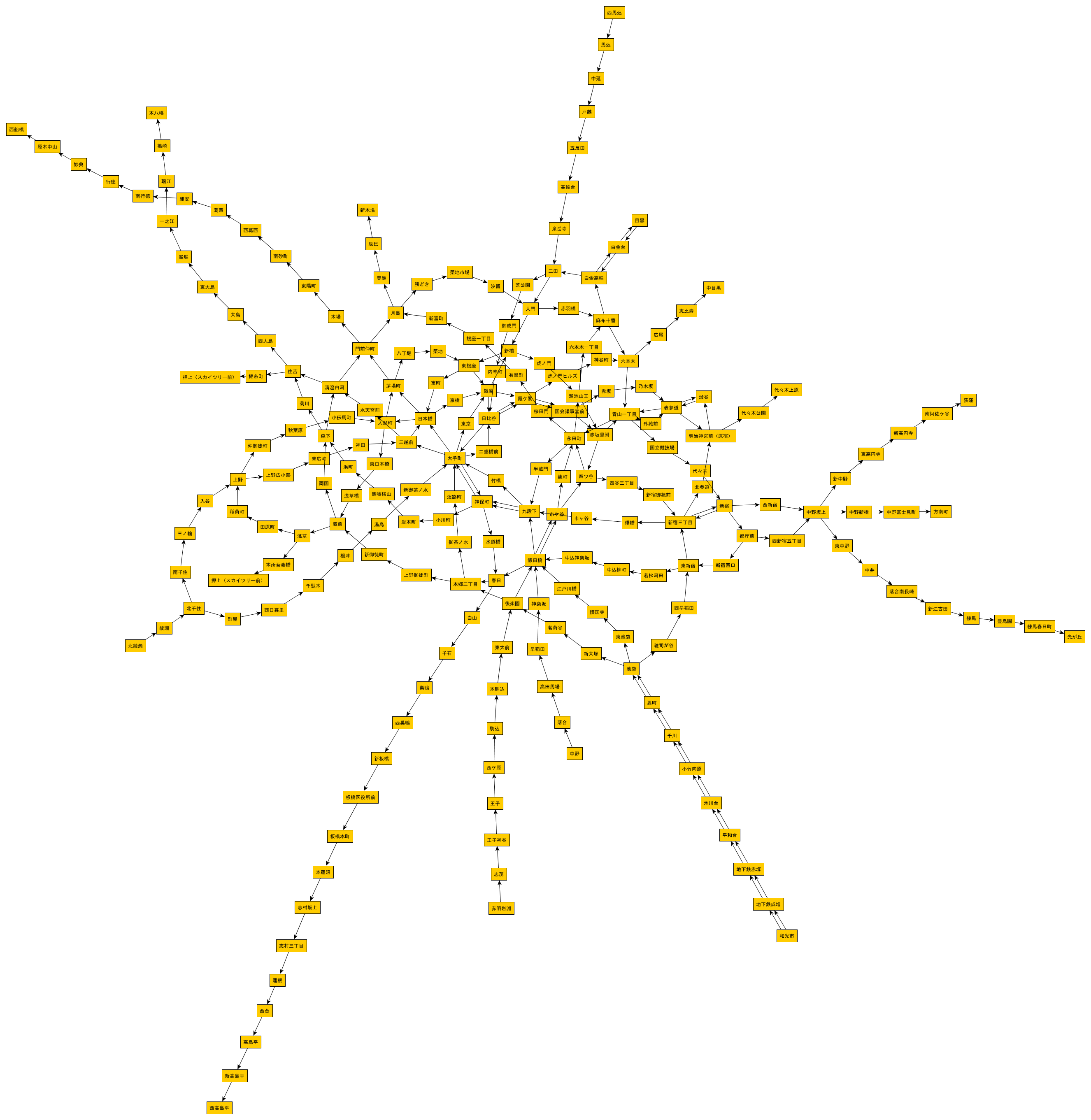# Integration of Ichigaya and Ichigaya

It looks like a route map, but there are two Ichigaya.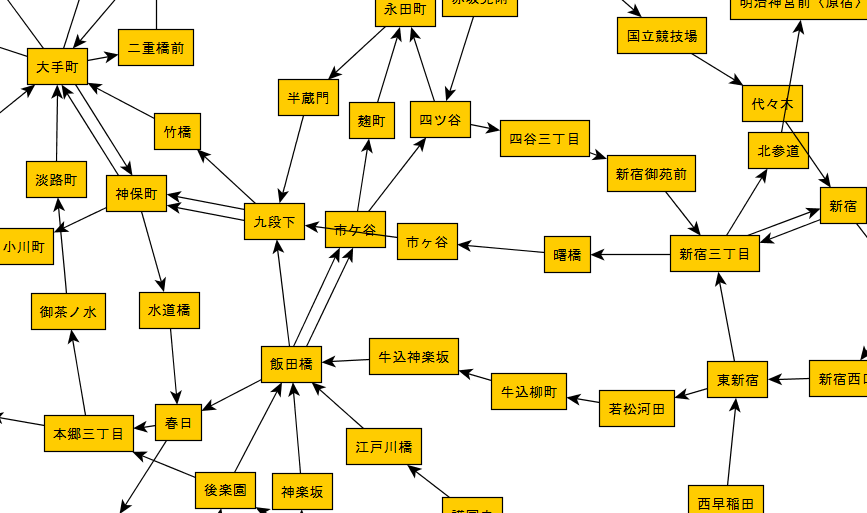If you look closely, you can see that there are two Ichigaya stations due to the difference between "ke" and "ga". If you look up Ichigaya Station on Wikipedia, This is written.

JR East and Tokyo Metro stations are referred to as "Ichigaya", and Toei Subway stations are referred to as "Ichigaya".

In the graph

• Akebonobashi → Ichigaya → Kudanshita (Toei Shinjuku Line)
• Iidabashi → Ichigaya → Yotsuya (Tokyo Metro Namboku Line)

Since it is, you can see that station data.jp accurately expresses this difference. To absorb this difference, change the reading of the station data described above as follows.

``````//Tokyo subway station
.filter(station -> lineCodes.contains(station.get(5)))
.map(station -> station.stream()
.map(item -> item.replace('Month', 'Ke')).collect(toList())) // 市Month谷と市Ke谷を統一
.collect(toList());
``````

Here, I decided to unify it to the Tokyo Metro style.

# Completed graph

The final form of the graph looks like this.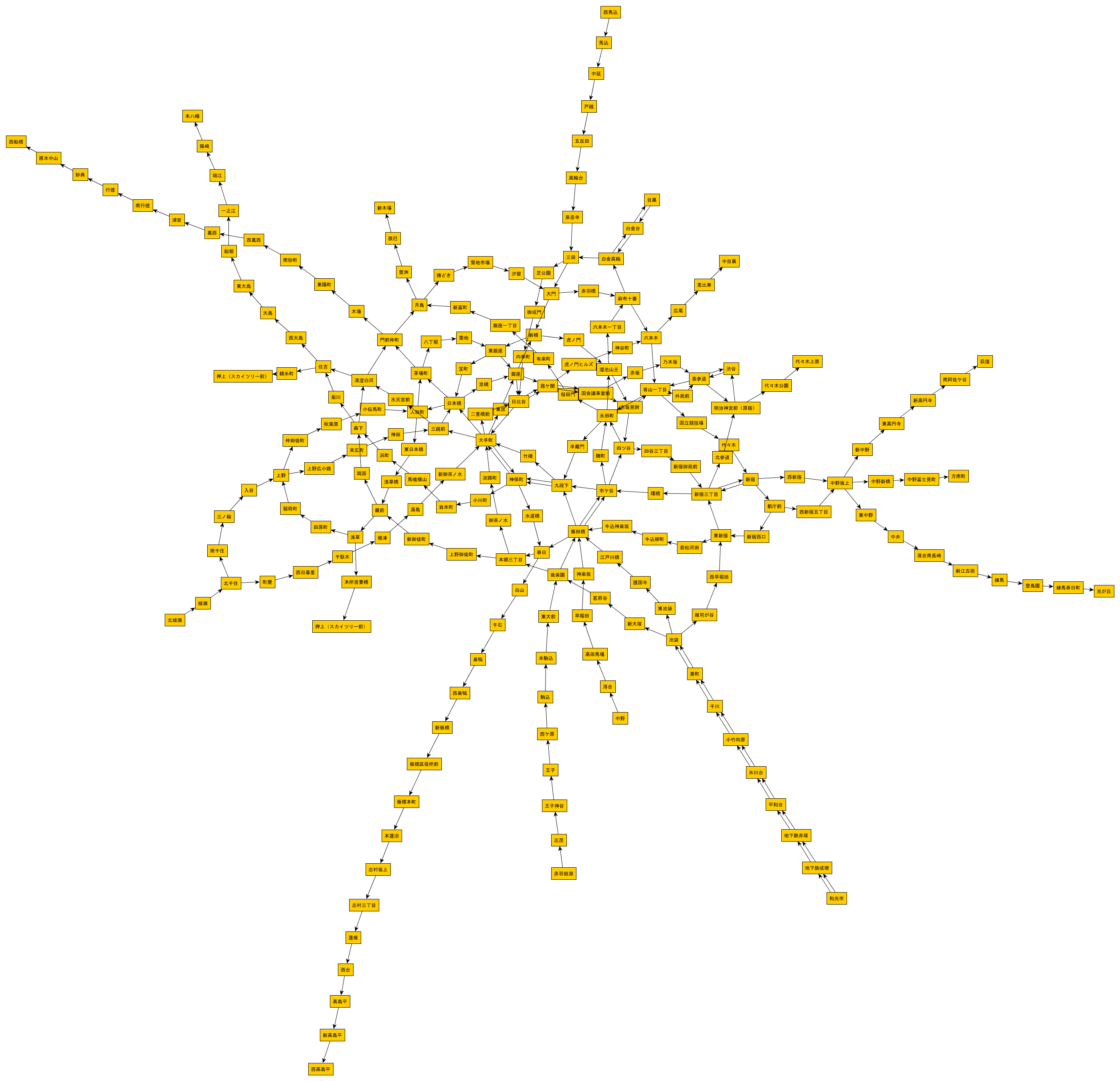# Summary

"Otemachi" is near the center, but if you look closely, you will find "Nishi-Funabashi" on the left end, "Ogikubo" on the right end, "Nishimagome" on the upper end, and "Nishi-Takashimadaira" on the lower end. It feels like the east and west and the north and south are reversed. It is unavoidable because the graph gives only topological information, but since the station data also includes the latitude and longitude information of the station, make a route map that is geographically accurate. Will be able to. GML node also has a color attribute, so you can color-code each line. yEd comes in a variety of layouts, so it's interesting to experiment with them. Most of the programs created this time use the Stream API. I found it very convenient because most of them can be described concisely with "one liner".